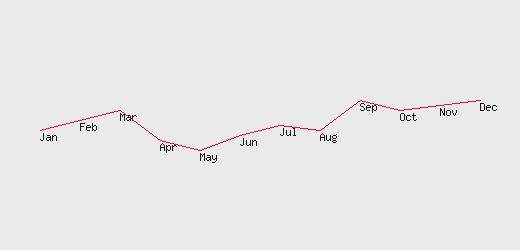# Plotting line graph by using data from MySQL table using PHP GD Support

We will try to learn how to draw ( or plot ) line graph using gd library and taking data from mysql table. Before starting this please read how to draw graphics lines tutorial.

## Reading data from the mysql table

To use line graph we are using data from mysql table. We will not discuss much on how to get data and there are many tutorials available here on how to connect and how to display data from the table. For our example we have taken table where monthly sales figure is stored. The table has two columns, one stores the month name and other stores sales figure of that month. We will plot a line graph taking the sales figure from the table. We have 12 rows for twelve months and data for sales figure for that month. You can download the database dump files at the end of this tutorial.

## Adjusting the Coordinates for the line graph

To plot the line graph we have to adjust the coordinates according to our data. In our graphics line tutorial we have learned that top left coordinate is 0,0 that mean horizontal axis known as X coordinate states from left edge of the screen and vertical axis known as Y coordinate starts from top line of the screen. To move to words right we have to increase the X coordinate value. As we have twelve months so to plot each month value we have to move fix distance horizontally so we will add some fixed width to move to next month. Let us call this horizontal gap as \$x_gap. We have twelve months so the graph width has to twelve times of this gap and will keep some left and right side gap. So we will take the total width of the graph as 13 times of the variable \$x_gap. Here is the code
``````
\$x_gap=50; // The gap between each point in y axis

\$x_max=\$x_gap*13; // Maximum width of the graph or horizontal axis
\$y_max=250; // Maximum  hight of the graph or vertical axis
``````
Here are some more points to be considered while drawing the graph.

One important point here is our zero on vertical axis starts from top. So if we want to plot the March month sale of 140 in the graph, then we have to subtract 140 from total height of the graph. So we will get the Y coordinate on the graph by subtracting the sales value for the month from the maximum height of the graph.

As we have to draw lines between two coordinates, we always have to store the previous point in memory and draw the line from previous point (memory) and the current value. So when we are reading data for the month of Sep we have to draw a line from Aug point to Sep point. After drawing the line from Aug to Sep we have to keep Sep sales figure in memory to draw line from Sep to Oct. This is achieved by shifting \$x2, \$y2 data to \$x1, \$y1 after drawing the line.

The first data should not start from the left most part of the image, or we can't start the image from \$x1=0 to \$x2=0 so we will set a flag at the beginning of the while loop and on the first time looping we will change again. This if condition will stop drawing the line for the first time inside the while loop.
``````
if(\$first_one=="no"){ // this is to prevent from starting \$x1= and \$y1=0
imageline (\$im,\$x1, \$y1,\$x2,\$y2,\$text_color); // Drawing the line between two points
}``````
Here is the output of this graphHere is the code of the graph
``````
<?php
include "db.php";

\$qt=mysql_query("select * from gd_graph");

\$x_gap=40; // The gap between each point in y axis

\$x_max=\$x_gap*13; // Maximum width of the graph or horizontal axis
\$y_max=250; // Maximum  hight of the graph or vertical axis
// Above two variables will be used to create a canvas of the image//

\$im = @ImageCreate (\$x_max, \$y_max);
or die ("Cannot Initialize new GD image stream");
\$background_color = ImageColorAllocate (\$im, 234, 234, 234);
\$text_color = ImageColorAllocate (\$im, 233, 14, 91);
\$graph_color = ImageColorAllocate (\$im,25,25,25);

\$x1=0;
\$y1=0;
\$first_one="yes";
while(\$nt=mysql_fetch_array(\$qt)){
//echo "\$nt[month], \$nt[sales]";
\$x2=\$x1+\$x_gap; // Shifting in X axis
\$y2=\$y_max-\$nt[sales]; // Coordinate of Y axis
ImageString(\$im,2,\$x2,\$y2,\$nt[month],\$graph_color);
//Line above is to print month names on the graph
if(\$first_one=="no"){ // this is to prevent from starting \$x1= and \$y1=0
imageline (\$im,\$x1, \$y1,\$x2,\$y2,\$text_color); // Drawing the line between two points
}
\$x1=\$x2; // Storing the value for next draw
\$y1=\$y2;
\$first_one="no"; // Now flag is set to allow the drawing
}
ImageJPEG (\$im);
?>``````
Download this script with sql dump to create your table.

This much is not enough for drawing line graphs. We have to take care of so many points. We can draw grid in the graph, write text on X and Y axis to mark different values. We can draw two line graphs with different coordinates in the same image, this is used for comparison. You can correlate sales to inventory level. We will try to learn all these in our next tutorial.
Line Chart Different types of graphs by using Google ChartsSubscribe to our YouTube Channel here

## Subscribe

* indicates required
Subscribe to plus2netplus2net.com

GD functions in PHP for handling images & graphics

How to check GD support in PHP
Header for an image in browser
Drawing of graphic lines using GD
Plotting line graph by drawing data from table
Adding vertical grid to line graph
Adding rectangles using imagerectangle()
Imagearc drawing arc and circle
Imagefilledarc drawing filled arc and circle
Resizing and adding border to Images
Script for Watermarking image on the fly using PHP GD
Generate image by using user input form data
 raja 29-12-2014 I dont now the mysql query using line chart

Post your comments , suggestion , error , requirements etc here